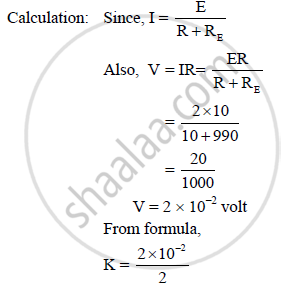HSC Science (Electronics) 12th Board ExamMaharashtra State Board
Share

# A Potentiometer Wire Has Length of 2m and Resistance 10ω. It is Connected in Series with Resistance 990ω and a Cell of E.M.F. 2v. Calculate the Potential Gradient Along the Wire. - HSC Science (Electronics) 12th Board Exam - Physics

#### Question

A potentiometer wire has length of 2m and resistance 10Ω. It is connected in series with resistance 990Ω and a cell of e.m.f. 2V. Calculate the potential gradient along the wire.

#### Solution

Given: L = 2 m, R = 10 Ω, RE = 990 Ω

E = 2 V

To find: Potential gradient of wire (K)

Formula: K = V/L∴ K = 10-2 V/m = 0.01 V/m

The potential gradient of wire is 0.01 V/m.

Is there an error in this question or solution?

#### APPEARS IN

2015-2016 (July) (with solutions)
Question 8.3 | 2.00 marks
Solution A Potentiometer Wire Has Length of 2m and Resistance 10ω. It is Connected in Series with Resistance 990ω and a Cell of E.M.F. 2v. Calculate the Potential Gradient Along the Wire. Concept: Meter Bridge.
S